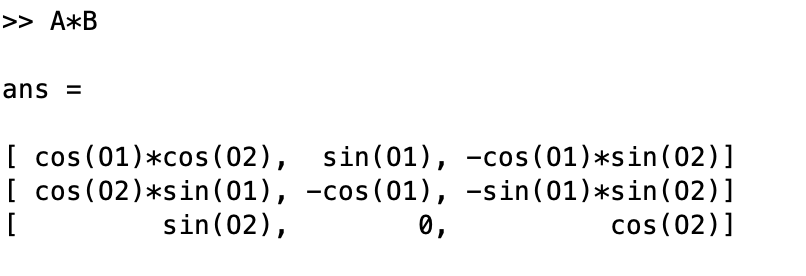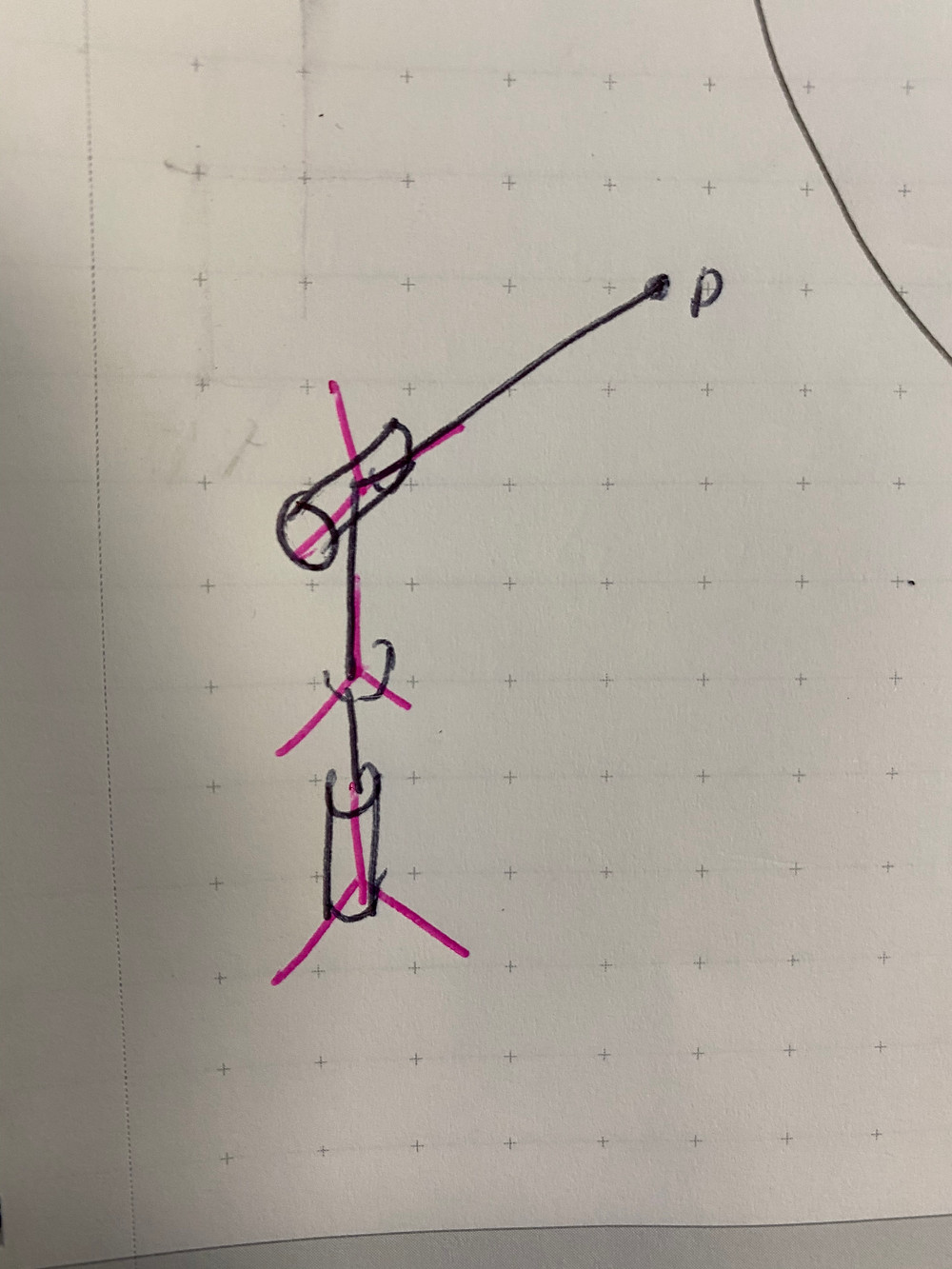Search
• aditya mehrotra.

# R matrices, 3D inverse-kinematics, denavit-hartenberg notation, and just all of robotics [learning]

In continuing the fashion of "you don't understand it until you can explain it," we're going to take the time to go through rotation matrices, 3D kinematics, denavit-hartenberg, and some other IK math for robots. All materials mostly come from Harry Asada's 2.12 class, full credit for explanations goes to him.

## transforming coordinate systems, rotation matrices in robots:

So the first thing that we need to understand before we get to any of these inverse kinematics and etc, is the idea of coordinate transforms from one origin/axis set to another, arbitrarily, in 3D space.

If we have a rigid body in 3-space, we care about two things, right? It's position, and it's orientation. The position can be defined by a single (x,y,z) coordinate of some point of interest on this rigid body. The problem isn' the location however, it's how do we describe orientation - that's a lot more complex.

The technique we will use to describe the rigid body in 3-space is we will create a new set of axis entirely. What will happen is the (x,y,z) point we chose for the location will become the origin of the new coordinate frame, and then we will create three new axis, the n, t, and b axis in place of the x, y, and z axis respectively.What does this mean for robotics, so we don't just get lost in math. If we look at a robot arm even with two degrees of freedom in 3D space, what this means is every time we have an actuated joint, or non-straight component of the robot, we'll have a new coordinate frame. In the diagram below, we have one frame for the robot base, then we have one frame for the next actuator which can rotate with respect to the base, and then we have one frame at the end for the end effector arm which can be rotated by the second actuator. So that's two degrees of freedom. (we will get more into how we placed these coordinate axis later)While this is a really trivial inverse kinematics problem, we'll still examine it for learning purposes. Let's say we have a point "P" is the end-effector of this robot arm (2DoF). And we want to find the coordinates of points P, given some rotation angles of the joints (ahem, forwards kinematics). What would we do?We want to find point "P" in terms of the BASE_FRAME of the robot, right? for arbitrary angles of Theta_1 and Theta_1, that's the forwards kinematics equations which can be solved inverse to get the Inverse_Kinematics of the system.

So now it turns out I can draw in a few vectors. Xbo, which is the x,y,z position of the next actuator with respect to the base of the robot. Xpb which is the location of point P with respect to the coordinate frame of the last actuator. And Xpo which is the location of point P with respect to the coordinate frame of the base of the robot. Do you see the difference in the diagram? Xpb would be something like distance*hat_j because in the case of the drawn diagram, point P will always lie on the y-axis of the coordinate frame of the second actuator.So now it turns out, some math can happen, and we won't really go through the derivation but the main idea, is all we are doing is vector addition. We can see the point of interest (P) from a certain coordinate frame (that of the actuator), and we can see the coordinate frame of the actuator's origin from the coordinate frame of the base. And we know the rotation angles between the frame of the origin and the frame of the base because we know the rotation of the actuators. So it turns out, the solution is as simple as this...Where "R" is the rotation matrix made of [n,t,b] column vectors which are the axis of the new coordinate frame as seen from the original coordinate frame as a function of Theta_1 and Theta_2. That's it! Also, these three vectors n, t, and b, are orthogonal to each other. Now we are going to describe how to do rotation matrices with a worked example. I'm still fuzzy on this myself so I'm going to write down everything I tried, and present the conclusions.So again, unless I'm doing math wrong, I believe you cannot create two independent rotation matrices and multiply them together as it reverses the direction of the Y-Axis in this case. It is possible I'm doing this wrong, I made a sign error/etc. If I did I'll come back and correct it after I ask the 2.12 TAs, but for security it might just be best to find n, t, and b with respect to both Theta_1 and Theta_2 in this example. This is also probably not a great example because in general, most of the time, the kinematics we will be doing involve only one rotation at a joint at a time, not two rotations at a joint. We could have split this half-way as well like the picture below and it would have probably made a lot more sense.This above diagram was probably the better way to attack this problem now that I look at it.

The last thing I want to mention about all of this is that if you have consecutive rotations, one after another, you just take the rotation from joint one to two, then two to three, then three to four, and keep adding the Rotation Matrix times the point of interest.

X = X0 + R1X1 + R1R2X2... so on...

## denavit-hartenberg notation:

Now I really want to spend some time on the Denvit-Hartenberg convention because it has become a robotics standard. It makes kinematics modeling much easier in deciding where to put your coordinate planes. (Where do I put the next coordinate frame?) If the question is, how do I place the coordinate frames on my robot so I may determine the rotation matrices and Forward/Inverse Kinematic Equations? Then Denavit Hartenberg is your answer. Here's a really good video on it: https://www.youtube.com/watch?v=rA9tm0gTln8

And here are my notes on it: We will get a tutorial up soon on how to use these on the CHIP robot itself.This is how we develop the coordinate frames themselves between actuators and then the above rotation matrix math is how we determine the kinematic equations.

In the next post, we'll do all of this through using the example of CHIP's leg. I'm excited!

1 view0 comments

### Recent Posts

See All

#### pcb notes: a general notes document on how to layout/design a PCB [random]

Here's a general notes document on how-to-layout a PCB. Putting it up here for future reference. #omgpcbs

#### Oleksandr Stepanenko: ok this dude's work is super cool [thingies]

© copyright 2019 | aditya mehrotra